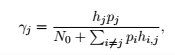# Problom presentation with CVX

Hello;

The following formula is part of a convex problem that I am trying to solve with CVX. However, I do not know how to present correctly the sum in the denominator. P is a column vector of size 3 and h_ij is a 3X3 matrix. The sum should be applied on column or raw only and it shall gives a vector of size 3. Each time I need to take only one value of the new vector. I need to show the value P because it is the optimization variable.

I appreciate any ideas or suggestion to correctly present the sum in the denominator.